# Software model of OpenNN

In this tutorial we present the software model of OpenNN. The whole process is carried out in the Unified Modeling Language (UML). The Unified Modeling Language (UML) is a general purpose visual modeling language that is used to specify, visualize, construct, and document the artifacts of a software system.

In order to construct a model for OpenNN, we follow a top-down development. This approach to the problem begins at the highest conceptual level and works down to the details. In this way, to create and evolve a conceptual class diagram, we iteratively model:

## 1. Classes

In colloquial terms a concept is an idea or a thing. In object-oriented modelling concepts are represented by means of classes. Therefore, a prime task is to identify the main concepts (or classes) of the problem domain. In UML class diagrams, classes are depicted as boxes.The next figure depicts a starting UML class diagram for the conceptual model of OpenNN.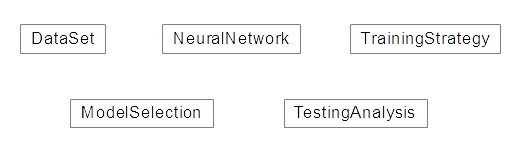Conceptual diagram for OpenNN.
• DataSet: The class that represents the concept of data set is called Dataset.
• NeuralNetwork: The class that represents the concept of neural network is called NeuralNetwork.
• TrainingStrategy: The class representing the concept of training strategy is called TrainingStrategy.
• ModelSelection: The class representing the concept of model selection is ModelSelection.
• TestingAnalysis: The class that represents the concept of analysis is called TestingAnalysis.

## 2. Associations

Once identified the main concepts in the model it is necessary to aggregate the associations among them. An association is a relationship between two concepts which points some significant or interesting information.

In UML class diagrams, an association is shown as a line connecting two classes. The appropriate associations among the main concepts of OpenNN are next identified to be included to the UML class diagram of the system: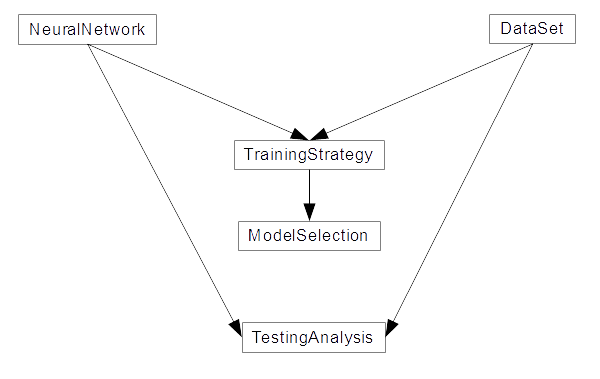• TrainingStrategy - NeuralNetwork & DataSet: TrainingStrategy handles Dataset and NerualNetwork to perform the training of a neural network.
• ModelSelection - TrainingStrategy: ModelSelection uses TrainingStrategy to check which model gets the best results.
• TestingAnalysis - DataSet & NeuralNetwork: TestingAnalisys evaluate NeuralNetwork over a DataSet.

## 3. Compositions

Classes are usually composed of another classes. The higher level classes manage the lower level ones. Regarding OpenNN, the concepts of DataSet, NeuralNetwork, TrainingStrategy, ModelSelection and TestingAnalysis, are quite high level structures. This means that this classes are composed by different elements.

In general the goal of OpenNN is to encapsulate basic concepts in elementary classes and then, create larger classes with broader concepts. For instance, referencing to NerualNetwork, PerceptronLayer is a subclass enclose in MultilayerPerceptron, this class represents the concept of a layer of perceptrons, and is necessary a set of layer to build a MultilayerPerceptron.

### DataSet

The following diagram shows the most relevant classes in DataSet: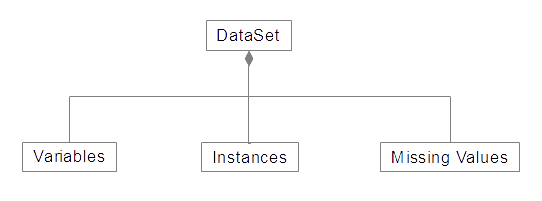• Variables: This class is used to store information about the variables of a data set.
• Instances: This class is used to store information about the instances of a data set.
• MissingValues: This class is used to store information about the missing values of a data set.

### NeuralNetwork

In the next uml diagram are the most important classes of NeuralNetwork: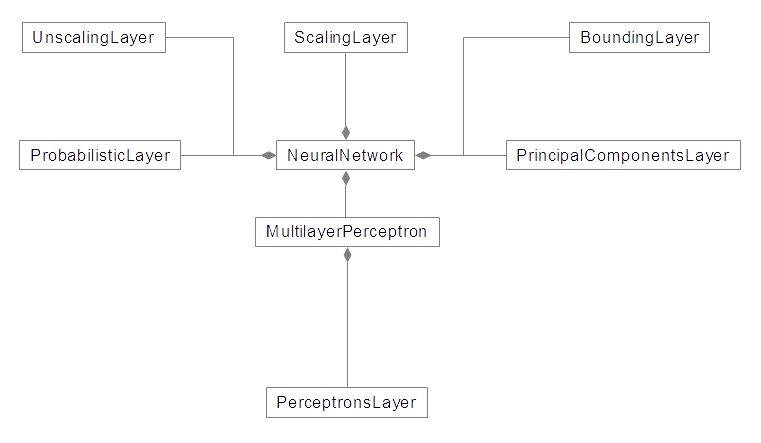• MultilayerPerceptron: This class represents the concept of multilayer perceptron.
• ScalingLayer: This class represents a layer of scaling neurons.
• UnscalingLayer: This class represents a layer of unscaling neurons.
• Boundinglayer: This class represents a layer of bounding neurons.
• ProbabilisticLayer: This class represents a layer of probabilistic neurons.
• PrincipalComponentsLayer: This class represents the layer of principal component analysis.

### TrainingStrategy

The following diagram shows the most relevant classes in TrainingStrategy: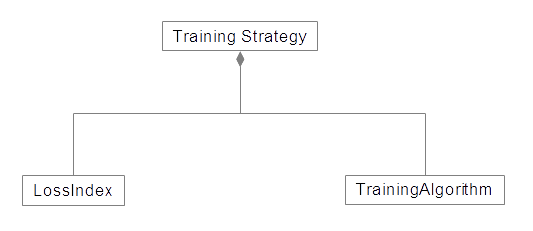• LossIndex: This abstract class represents the concept of error term.
• TrainingAlgorithm: This abstract class represents the concept of training algorithm for a neural network.

### ModelSelection

In the picture below, the most important classes of ModelSelection are shown: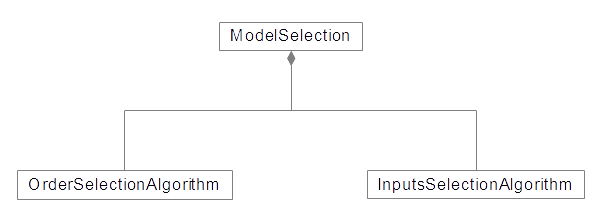• InputSelectionAlgorithm: This abstract class represents the concept of inputs selection algorithm for a neural network.
• OrderSelectionAlgorithm: This abstract class represents the concept of order selection algorithm for a neural network.

## 4. Derived classes

In object-oriented programming, some classes are designed only as a parent from which sub-classes may be derived, but which is not itself suitable for instantiation. This is said to be an abstract class, as opposed to a concrete class, which is suitable to be instantiated.

The derived class contains all the features of the base class, but may have new features added or redefine existing features. Associations between a base class an a derived class are of the kind is a.

### TrainingStrategy

The classes: Mean Square error, CrossEntropyError, MinkowskiError, NormalizedSquaredError, RootMeanSquareError, WeightedSquareError are derived from LossIndex, all of them have in common that they inherit the characteristics of the base class, however each of this classes also introduce new features such us its own definition of error.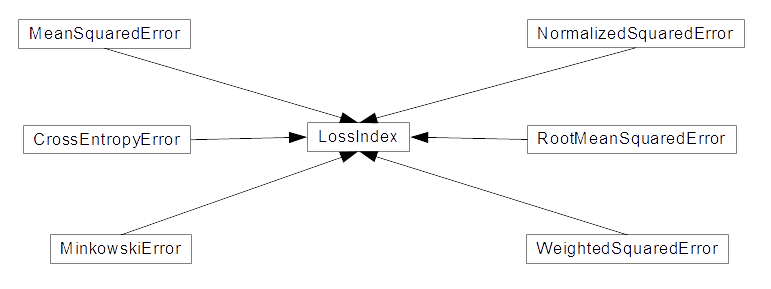Likewise GradientDescent, Adam, ConjugateGradient, QuasiNewtonMethod, LevenbergMarquadtAlgorithm are a set of concrete classes that have inherited all the characteristics of the abstract class TrainingAlgotihm.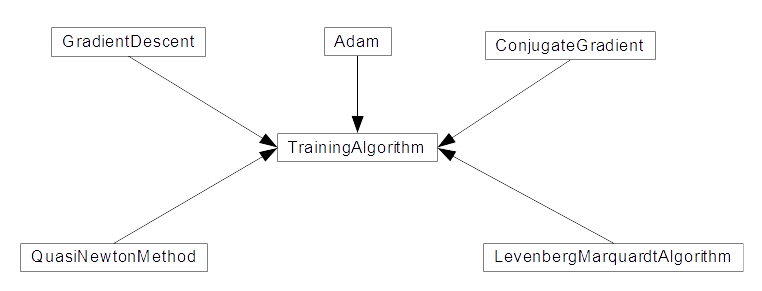### ModelSelection

Like TrainingStrategy, ModelSelection is composed by 2 abstract classes InputSelectionAlgorithm and OrderSelectionAlgorithm, both have their own derived classes that inherit the features of each one.

InputSelectionAlgorithm is an abstract class composed by 3 concretes classes: GrowingInputs, GeneticAlgorithm, PruningInputs.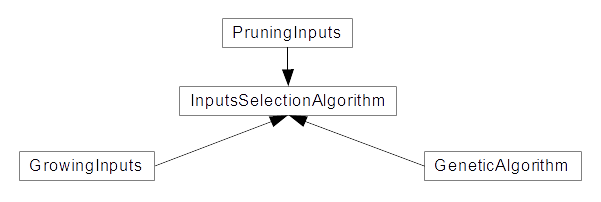OrderSelectionAlgorithm is an abstract class composed by 3 concretes classes: GoldenSelectionOrder, SimulatedAnnealingOrder, IncrementalOrder.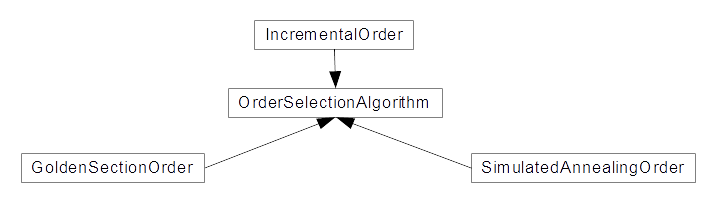## 5. Members and methods

A member (or attribute) is a named value or relationship that exists for all or some instances of a class. A method (or operation) is a procedure associated with a class.

In UML class diagrams, classes are depicted as boxes with two sections: the top that lists the attributes of the class, and the bottom that lists the operations. The main members and methods of the different OpenNN classes are described throughout all this manual.

The most important attributes and methods of the DataSet class: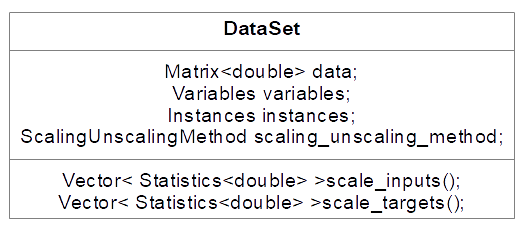The most important attributes and methods of NeruralNetwork class are: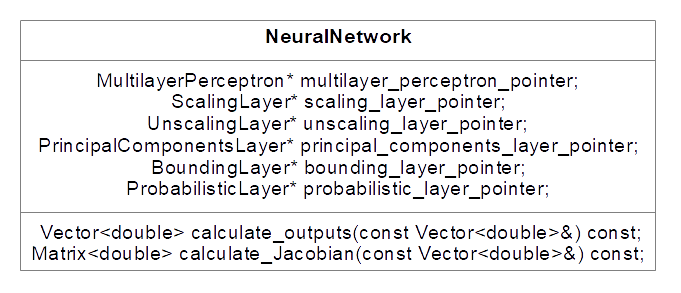In the following chart the most important attributes of the training strategy class are shown: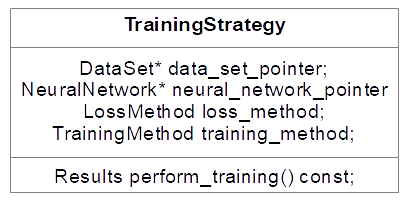The most important attributes and methods of the ModelSelection class are: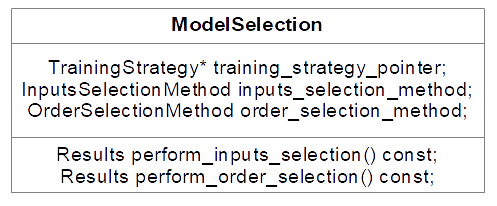The next box shows the most important attributes and methods of TestingAnalisys class:## Bibliography

• C. Bishop. Neural Networks for Pattern Recognition. Oxford University Press, 1995.
• H. Demuth, M. Beale, and M. Hagan. Neural Network Toolbox User's Gide. The MathWorks, Inc., 2009.
• S. Haykin. Neural Networks: A Comprehensive Foundation. Prentice Hall.
• R. Lopez. Neural Networks for Variational Problems in Engineering. PhD Thesis, Technical University of Catalonia, 2008.